Descriptive Statistics

# 9 Measures of the Center of the Data

The “center” of a data set is also a way of describing location. The two most widely used measures of the “center” of the data are the mean (average) and the median. To calculate the mean weight of 50 people, add the 50 weights together and divide by 50. Technically this is the arithmetic mean. We will discuss the geometric mean later. To find the median weight of the 50 people, order the data and find the number that splits the data into two equal parts meaning an equal number of observations on each side. The weight of 25 people are below this weight and 25 people are heavier than this weight. The median is generally a better measure of the center when there are extreme values or outliers because it is not affected by the precise numerical values of the outliers. The mean is the most common measure of the center.

NOTE

The words “mean” and “average” are often used interchangeably. The substitution of one word for the other is common practice. The technical term is “arithmetic mean” and “average” is technically a center location. Formally, the arithmetic mean is called the first moment of the distribution by mathematicians. However, in practice among non-statisticians, “average” is commonly accepted for “arithmetic mean.”

When each value in the data set is not unique, the mean can be calculated by multiplying each distinct value by its frequency and then dividing the sum by the total number of data values. The letter used to represent the sample mean is an x with a bar over it (pronounced “x bar”):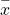.

The Greek letter μ (pronounced “mew”) represents the population mean. One of the requirements for the sample mean to be a good estimate of the population mean is for the sample taken to be truly random.

To see that both ways of calculating the mean are the same, consider the sample:
1; 1; 1; 2; 2; 3; 4; 4; 4; 4; 4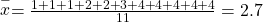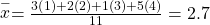In the second calculation, the frequencies are 3, 2, 1, and 5.

You can quickly find the location of the median by using the expression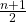.

The letter n is the total number of data values in the sample. If n is an odd number, the median is the middle value of the ordered data (ordered smallest to largest). If n is an even number, the median is equal to the two middle values added together and divided by two after the data has been ordered. For example, if the total number of data values is 97, then=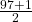= 49. The median is the 49th value in the ordered data. If the total number of data values is 100, then=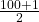= 50.5. The median occurs midway between the 50th and 51st values. The location of the median and the value of the median are not the same. The upper case letter M is often used to represent the median. The next example illustrates the location of the median and the value of the median.

AIDS data indicating the number of months a patient with AIDS lives after taking a new antibody drug are as follows (smallest to largest):
3; 4; 8; 8; 10; 11; 12; 13; 14; 15; 15; 16; 16; 17; 17; 18; 21; 22; 22; 24; 24; 25; 26; 26; 27; 27; 29; 29; 31; 32; 33; 33; 34; 34; 35; 37; 40; 44; 44; 47;
Calculate the mean and the median.

The calculation for the mean is: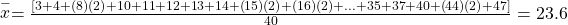To find the median, M, first use the formula for the location. The location is: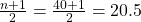Starting at the smallest value, the median is located between the 20th and 21st values (the two 24s):
3; 4; 8; 8; 10; 11; 12; 13; 14; 15; 15; 16; 16; 17; 17; 18; 21; 22; 22; 24; 24; 25; 26; 26; 27; 27; 29; 29; 31; 32; 33; 33; 34; 34; 35; 37; 40; 44; 44; 47;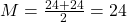Suppose that in a small town of 50 people, one person earns ?5,000,000 per year and the other 49 each earn ?30,000. Which is the better measure of the “center”: the mean or the median?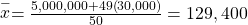M = 30,000

(There are 49 people who earn ?30,000 and one person who earns ?5,000,000.)

The median is a better measure of the “center” than the mean because 49 of the values are 30,000 and one is 5,000,000. The 5,000,000 is an outlier. The 30,000 gives us a better sense of the middle of the data.

Another measure of the center is the mode. The mode is the most frequent value. There can be more than one mode in a data set as long as those values have the same frequency and that frequency is the highest. A data set with two modes is called bimodal.

Statistics exam scores for 20 students are as follows:

5053595963637272727272767881838484849093

Find the mode.

The most frequent score is 72, which occurs five times. Mode = 72.

Five real estate exam scores are 430, 430, 480, 480, 495. The data set is bimodal because the scores 430 and 480 each occur twice.

When is the mode the best measure of the “center”? Consider a weight loss program that advertises a mean weight loss of six pounds the first week of the program. The mode might indicate that most people lose two pounds the first week, making the program less appealing.

NOTE

The mode can be calculated for qualitative data as well as for quantitative data. For example, if the data set is: red, red, red, green, green, yellow, purple, black, blue, the mode is red.

### Calculating the Arithmetic Mean of Grouped Frequency Tables

When only grouped data is available, you do not know the individual data values (we only know intervals and interval frequencies); therefore, you cannot compute an exact mean for the data set. What we must do is estimate the actual mean by calculating the mean of a frequency table. A frequency table is a data representation in which grouped data is displayed along with the corresponding frequencies. To calculate the mean from a grouped frequency table we can apply the basic definition of mean: mean =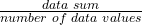We simply need to modify the definition to fit within the restrictions of a frequency table.

Since we do not know the individual data values we can instead find the midpoint of each interval. The midpoint is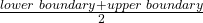. We can now modify the mean definition to be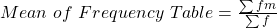where f = the frequency of the interval and m = the midpoint of the interval.

A frequency table displaying professor Blount’s last statistic test is shown. Find the best estimate of the class mean.

50–56.5 1
56.5–62.5 0
62.5–68.5 4
68.5–74.5 4
74.5–80.5 2
80.5–86.5 3
86.5–92.5 4
92.5–98.5 1
• Find the midpoints for all intervals
50–56.5 53.25
56.5–62.5 59.5
62.5–68.5 65.5
68.5–74.5 71.5
74.5–80.5 77.5
80.5–86.5 83.5
86.5–92.5 89.5
92.5–98.5 95.5
• Calculate the sum of the product of each interval frequency and midpoint.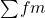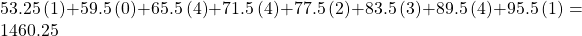•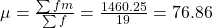Try It

Maris conducted a study on the effect that playing video games has on memory recall. As part of her study, she compiled the following data:

Hours teenagers spend on video games Number of teenagers
0–3.5 3
3.5–7.5 7
7.5–11.5 12
11.5–15.5 7
15.5–19.5 9

What is the best estimate for the mean number of hours spent playing video games?

Find the midpoint of each interval, multiply by the corresponding number of teenagers, add the results and then divide by the total number of teenagers
The midpoints are 1.75, 5.5, 9.5, 13.5,17.5.
Mean = (1.75)(3) + (5.5)(7) + (9.5)(12) + (13.5)(7) + (17.5)(9) = 409.75/38 = 10.78

### References

Data from The World Bank, available online at http://www.worldbank.org (accessed April 3, 2013).

“Demographics: Obesity – adult prevalence rate.” Indexmundi. Available online at http://www.indexmundi.com/g/r.aspx?t=50&v=2228&l=en (accessed April 3, 2013).

### Chapter Review

The mean and the median can be calculated to help you find the “center” of a data set. The mean is the best estimate for the actual data set, but the median is the best measurement when a data set contains several outliers or extreme values. The mode will tell you the most frequently occuring datum (or data) in your data set. The mean, median, and mode are extremely helpful when you need to analyze your data, but if your data set consists of ranges which lack specific values, the mean may seem impossible to calculate. However, the mean can be approximated if you add the lower boundary with the upper boundary and divide by two to find the midpoint of each interval. Multiply each midpoint by the number of values found in the corresponding range. Divide the sum of these values by the total number of data values in the set.

### Formula Review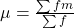Where f = interval frequencies and m = interval midpoints.

The arithmetic mean for a sample (denoted by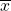) is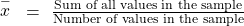The arithmetic mean for a population (denoted by μ) is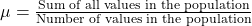### Find the mean for the following frequency tables.GradeFrequency49.5–59.5259.5–69.5369.5–79.5879.5–89.51289.5–99.55Daily low temperatureFrequency49.5–59.55359.5–69.53269.5–79.51579.5–89.5189.5–99.50Points per gameFrequency49.5–59.51459.5–69.53269.5–79.51579.5–89.52389.5–99.52

Use the following information to answer the next three exercises: The following data show the lengths of boats moored in a marina. The data are ordered from smallest to largest: 161719202021232425252526262727272829303233333435373940

Calculate the mean.

Mean: 16 + 17 + 19 + 20 + 20 + 21 + 23 + 24 + 25 + 25 + 25 + 26 + 26 + 27 + 27 + 27 + 28 + 29 + 30 + 32 + 33 + 33 + 34 + 35 + 37 + 39 + 40 = 738;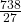= 27.33

Identify the median.

Identify the mode.

The most frequent lengths are 25 and 27, which occur three times. Mode = 25, 27

Use the following information to answer the next three exercises: Sixty-five randomly selected car salespersons were asked the number of cars they generally sell in one week. Fourteen people answered that they generally sell three cars; nineteen generally sell four cars; twelve generally sell five cars; nine generally sell six cars; eleven generally sell seven cars. Calculate the following:

sample mean == _______

median = _______

4

mode = _______

### Homework

The most obese countries in the world have obesity rates that range from 11.4% to 74.6%. This data is summarized in the following table.

Percent of population obese Number of countries
11.4–20.45 29
20.45–29.45 13
29.45–38.45 4
38.45–47.45 0
47.45–56.45 2
56.45–65.45 1
65.45–74.45 0
74.45–83.45 1
1. What is the best estimate of the average obesity percentage for these countries?
2. The United States has an average obesity rate of 33.9%. Is this rate above average or below?
3. How does the United States compare to other countries?

(Figure) gives the percent of children under five considered to be underweight. What is the best estimate for the mean percentage of underweight children?

Percent of underweight children Number of countries
16–21.45 23
21.45–26.9 4
26.9–32.35 9
32.35–37.8 7
37.8–43.25 6
43.25–48.7 1

The mean percentage,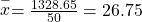### Bringing It Together

Javier and Ercilia are supervisors at a shopping mall. Each was given the task of estimating the mean distance that shoppers live from the mall. They each randomly surveyed 100 shoppers. The samples yielded the following information.

Javier Ercilia6.0 miles 6.0 miles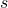4.0 miles 7.0 miles
1. How can you determine which survey was correct ?
2. Explain what the difference in the results of the surveys implies about the data.
3. If the two histograms depict the distribution of values for each supervisor, which one depicts Ercilia’s sample? How do you know?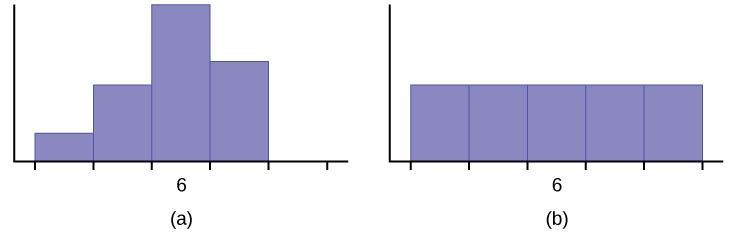Use the following information to answer the next three exercises: We are interested in the number of years students in a particular elementary statistics class have lived in California. The information in the following table is from the entire section.

Number of years Frequency Number of years Frequency
Total = 20
7 1 22 1
14 3 23 1
15 1 26 1
18 1 40 2
19 4 42 2
20 3

What is the IQR?

1. 8
2. 11
3. 15
4. 35

a

What is the mode?

1. 19
2. 19.5
3. 14 and 20
4. 22.65

Is this a sample or the entire population?

1. sample
2. entire population
3. neither

b

### Key Terms

Frequency Table
a data representation in which grouped data is displayed along with the corresponding frequencies
Mean (arithmetic)
a number that measures the central tendency of the data; a common name for mean is ‘average.’ The term ‘mean’ is a shortened form of ‘arithmetic mean.’ By definition, the mean for a sample (denoted by) is, and the mean for a population (denoted by μ) is.
Mean (geometric)
a measure of central tendency that provides a measure of average geometric growth over multiple time periods.
Median
a number that separates ordered data into halves; half the values are the same number or smaller than the median and half the values are the same number or larger than the median. The median may or may not be part of the data.
Midpoint
the mean of an interval in a frequency table
Mode
the value that appears most frequently in a set of data